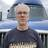Create x number of records with button

I hate two date fields, and a formula to calculate the total # of days between them (x).

I then want to have a button that when clicked, will create x number of records in a composition table.

Is that possible?Yes, you can use a for-loop to create x numer of records.

`for i from 0 to x do`
`create YourTableName`
`end`

Thanks Sean!

The next question is, say I have a range range of the 1st to the 9th of the month. I have a formula to calculate the number of days (9) which I can then use to create the records in the composition table... those records have a date field, and will need to be each day of the date range.

Is there a way to have it auto populate those dates?

I'm assuming it would be something along the lines of having the first record take the start date of the parent record, then telling the other dates to add a day to the previous records date?Hi Sarah, You're on the right track. You can use something like the following to update the date field...

`for i from 0 to x do`
`let newRecord := (create YourTableName);`
`newRecord.(Date := today() + i)`
`end`